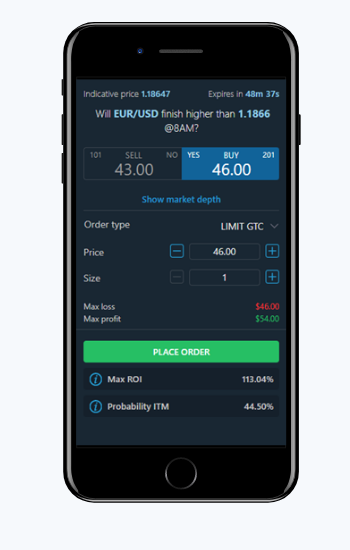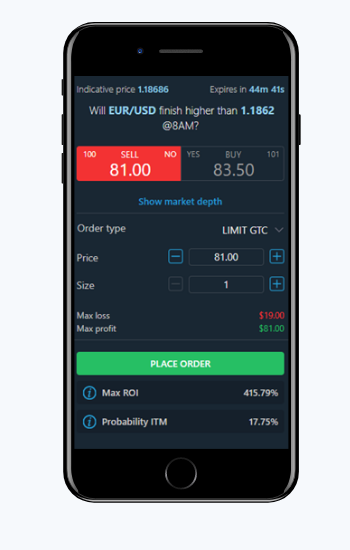# Max ROI

The maximum ROI is calculated using the best current bid or offer price at a particular moment and assumes you hold the contract until expiration to receive the full \$100 payout per contract, minus the cost of your trade and fees. Nadex provides no guarantee of these returns and assumes no liability for trading decisions based on this Maximum ROI tool. Any trading decisions you make are solely your responsibility and at your own risk.

The Max ROI formula is calculated as follows:

(Gain - Cost) / Cost = Result x 100 = ROI

((\$100 Payout - \$2.00 round trip fees) - Cost of Trade) / Cost of Trade = Result x 100 = ROI

Let's look at a buy-side example. In this scenario, you are buying a contract for \$46.00 and if you are correct at expiration, you will receive \$100.00 minus \$2.00 for the roundtrip fees.

Therefore, your Max ROI if you hold the contract until expiration and the contract settles in your favor is 113.04%.

= ((100 – 2) – 46) / 46

= (98 – 46) / 46

= 1.1304 x 100

= 113.04%In the sell-side example below, you are entering the contract at \$81.00, meaning your cost is \$19.00.

Therefore, your Max ROI if you hold the contract until expiration and the contract settles in your favor is 415.79%.

= ((100 – 2) – (100 – 81)) / (100 – 81)

= (98 – 19) / 19

= 4.15789 x 100

= 415.79%As always, please reach out to customerservice@nadex.com if you have any questions!